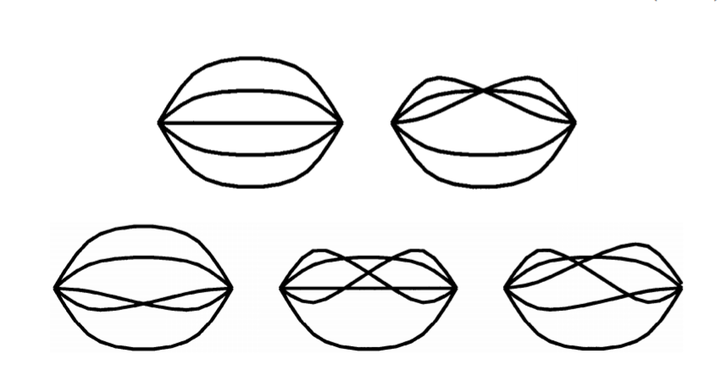# Λ (1405) as a multiquark state### Abstract

In the QCD sum rule approach we predict the Λ (1405) mass by choosing the π0Σ0 multiquark interpolating field. It is found that the mass is about 1.419 GeV from Π1 (q 2) sum rule which is more reliable than Πq (q 2) sum rule, where Πq (q 2) and Π1 (q 2) are two invariant functions of the correlator Π (q 2). We also present the sum rules for the K + p and the π+Σ+ multiquark states, and compare to those for the π0Σ0 multiquark state. The mass of the Λ (1600) can be also reproduced in our approach.

Publication
The Eur. Phys. J. A-Hadrons and Nuclei